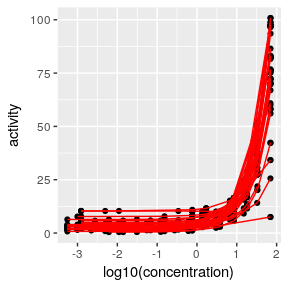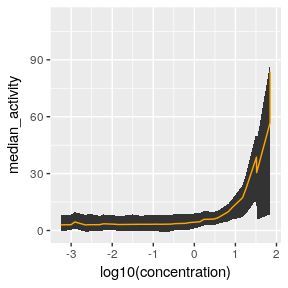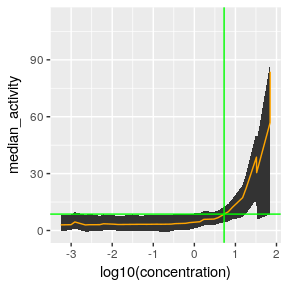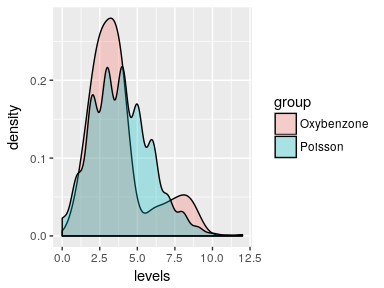# Concentration-Response Analysis to Identify a Chemical’s Point of Departure Using the AOP Package

#### Monday, August 24, 2015

This vignette describes how to use the aop package to calculate a safe margin of exposure for potential oxybenzone endocrine disruptor activity via activation of the estrogen receptor (ESR1). This analysis uses concentration-response data to identify a chemical’s point of departure (POD). Then, additional exposure and skin absorption data are used to derive a safe margin of exposure.

## Background

In chemical risk assessment, the POD is the concentration/dose at which a population begins to experience an adverse outcome. Mathematically, this can be thought of as the point at which the population measurably departs from the background rate of adverse outcome occurence. For instance, consider that there is a background rate of heart disease, even in the absence of a chemical exposure. In chemical risk assessment, we are interested in finding that chemical exposure concentration/dose at which the population begins to deviate from that background rate of heart disease.

In many instances, we observe that these concentration/dose-response curves have a sigmoidal shape, with an asymptote at the lower concentrations/doses. This asymptote represents the background rate of the adverse outcome. The POD, as calculated in this package, is based on the concept of identifying the point at which the curve begins to deviate from the asympototic region. We identify this by calculating the point at which the median of the concentration/dose-response curve intersects with the 95% confidence envelope for the asymptote. This is the point at which the median of the population has met the 95% confidence envelope of the asymptote, and thus reflects the movement of the population away from the asymptotic region.

This method has advantages over the commonly used benchmark dose analysis as it does not make assumptions about the possible models, it is data-driven, and does not require the use of specific models which may or may not be appropriate for the data. This bootstrap metaregression analysis approach allows the data to speak for itself.

## Example: Calculating the POD for Oxybenzone Estrogen Receptor Activation

In this example, we will identify the POD for the activation of the estrogen receptor by oxybenzone using Tox21 data. This analysis will allow us to identify a screening level concentration and a potential margin of exposure for risk screening assessments.

The oxybenzone dataset consists of two different assays from PubChem: AID 743075 (Substance ID: 144209183, Chemical ID: 4632) and AID 743079 (Substance ID: 144203969, Chemical ID: 4632). There are 58 rows and 4 columns/variables.

This code will read in the oxybenzone data and perform a bootstrap metaregression. There are 15 concentrations in the dataset that we want to sample from (hence, the 15 as the second parameter). We want to run the bootstrap metaregression 1,000 times. This will create a bootstrap_metaregression object, bmr.

library(aop)
set.seed(1332142)
bmr_obj <- bootstrap_metaregression(oxybenzone, 15, 1000)

The bmr object contains the models, the predicted values (model fits or fits), the median values for the median concentration-response curve across all of the simulations, and the confidence envelope across all of the simulated concentration-response curves.

The bmr object is used for downstream analysis and visualizations. For instance, we can look at the spaghetti plot of a selection of the models using this code:

library(ggplot2)
plot_metaregression_spaghetti_plot(bmr_obj, number_to_plot=40)We can also visualize the confidence envelope and median using this plot

plot_metaregression_confidence_envelope(bmr_obj, graph_pod = FALSE)To identify the point of departure, we need to perform a slope analysis (to identify our slope threshold), and then use that information to identify the POD. Here’s the code to do that and to visualize the result:

slope_pod <- slope_pod_analysis(bmr_obj, 0.0001, 10, 0.1)
pod_and_threshold <- pod_envelope_analysis(bmr_obj, slope_pod, 10, min(oxybenzone$Concentration), max(oxybenzone$Concentration), 0.1)
plot_metaregression_confidence_envelope(bmr_obj, graph_pod = TRUE, pod = pod_and_threshold$pod, pod_threshold=pod_and_threshold$threshold)The paramters for the slope_pod_analysis function are the bmr object, bmr_obj, the lower and upper bound for the region to interpolate, and the step or increment size. So, this function will interpolate response values for the concentrations from 0.0001 through 10, with 0.1 unit increments.

The pod_envelope_analysis function identifies the point of departure and the threshold value based on the slope analysis. The parameters include the bmr object, the slope_pod_analysis object, the slope threshold value, the lower and upper interpolation range, and the increment size. Generally, the lower and upper interpolation ranges should be the full range of the concentration-response analysis.

The next function, plot_metaregression_confidence_envelope, plots the confidence envelope and median, as well as crosshairs that allow the user to visualize where the threshold response value is and the location of the POD.

The user can see what the threshold and the POD are by doing:

pod_and_threshold
##        pod threshold
## 1 5.300556   8.63351

Because the POD is based on in vitro assays, the POD represents an internal point of departure, meaning it represents a likely blood level. Since oxybenzone exposure is largely as a result of sunscreen products applied to the skin, oxybenzone is not likely to undergo first-pass metabolism in the liver. Thus, skin absorption levels are likely to directly influence blood levels, and then the oxybenzone is likely to be metabolized as the blood travels through the liver.

Based on Gonzalez et al, 2006, 1.2-8.7% of the administered oxybenzone is absorbed by the skin. Based on Figure 1 in their paper, I have estimated the following values for % of administered oxybenzone that is found in the urine:

oxybenzone_levels <- c(1.2, 1.5, 2, 2.2, 2.3, 2.3, 2.3, 2.7, 2.8, 3.0, 3.0, 3.5, 3.5, 3.6, 3.7, 3.8, 4.0, 4.1, 4.1, 6.0, 7.1, 8.0, 8.7)

This fits the following Poisson distribution rather well. For instance, a Poisson distribution has a mean that equals the variance. In this case, the mean of the oxybenzone_level is 3.9 and the variance is 3.90, so although not an exact fit, it’s not too bad. I prefer a Poisson distribution using the empirical variance in this case, as opposed to the empirical mean, as I want to incorporate more uncertainty in the distribution.

oxybenzone_df <- data.frame(levels = oxybenzone_levels, group="Oxybenzone")
r_levels <- data.frame(levels = rpois(2000, var(oxybenzone_levels)), group = "Poisson")
oxybenzone_stuff <- rbind(oxybenzone_df, r_levels)

ggplot(oxybenzone_stuff, aes(x=levels, fill=group)) +
geom_density(alpha=.3)Based on this information, and by making some assumptions, we can use the Poisson distribution to make some screening level assessments using the margin of exposure (MOE) approach. The MOE can be thought of as a safety margin on the external dose, using the internal POD as its basis. For instance, if the external dose that corresponds to the internal POD is 10mg, and we want to have a 100x margin of safety, then the screening exposure level is 10mg / 100 = 0.1mg or 100ug.

Note that this approach assumes the following:

• The amount of oxybenzone available in the blood is either not bound to proteins, or will freely dissociate from plasma proteins in the target tissue
• Since we lack information on the relevance of oxybenzone metabolites in activating the estrogen receptor, we will assume that metabolism is irrelevant for toxicity (i.e., oxybenzone and its conjugated metabolites have similar estrogen receptor activation potential)
• Repeated, at least daily, administration of oxybenzone such that steady state is achieved. In the Gonzalez et al [#1] study steady state is achieved, with demonstration of oxybenzone accumulation, by day 3.

We know that the POD is approximately 5.3uM (may be slightly different due to simulation). We also know that the population’s blood levels of oxybenzone, by percent administration, follows a Poisson distribution, and that oxybenzone’s molecular weight is 228.24 g/mol. If we assume that humans have approximately 5L of blood, then we can back-calculate the external applied dose of oxybenzone that results in the POD.

oxybenzone_g_per_L <- 228.24 * pod_and_threshold
oxybenzone_total_blood <- oxybenzone_g_per_L * 5
if(qpois(1-0.005, 3.9, lower.tail=FALSE) != 0){
administered_population_lower <- oxybenzone_total_blood / qpois(1-0.005, 3.9, lower.tail=FALSE)
} else{
}
administered_population_higher <- oxybenzone_total_blood / qpois(1-0.995, 3.9, lower.tail=FALSE)
administered_population_lower
##  0
administered_population_higher
##        pod
## 1 604.8994

However, if we’re interested in protecting a larger segment of the population, such as those who might absorb abnormally high amounts of oxybenzone through their skin, such as those who are at the 1:10,000 tail of the distribution, then we would calculate the external applied dose for that group as:

administered_population_1_in_10000 <- oxybenzone_total_blood / qpois(1-0.99995, 3.9, lower.tail=FALSE)

And now the margin of exposure for the largest part of the population would be 6.05g:

administered_population_higher / 100
##        pod
## 1 6.048994

While the margin of exposure that was protective of most of the population, and likely to include sensitive populations, would be 4.32g:

administered_population_1_in_10000 / 100
##       pod
## 1 4.32071

Thus, if I were a risk assessor, and was concerned about the potential estrogenic effects of oxybenzone, and I had no way to differentiate a sensitive from less sensitive population, I would use the 4.32g margin of exposure as a screening level. If the population as a whole were potentially exposed at this level, I would want to perform a risk assessment. If sufficient data were not available for a risk assessment, then I would suggest appropriate studies should be performed.

According to the Mayo Clinic (Accessed 24-Aug-2015) and the American Academy of Dermatology it takes about 1oz of sunscreen to cover all exposed parts on the human body. That is approximately 28g. If using Banana Boat Sunscreen Sport Performance Broad Spectrum Sun Care Sunscreen Lotion - SPF 30, 8 Ounce available at Amazon, then we know that 4% of the applied product is oxybenzone. If 28g of the product is used, then we know that we will be exposed to 1.12g during the first exposure. If we go swimming, and have to reapply, then we know we are now exposed to 2.24g. As each application will generally result in 1.12g of exposure, I estimate that it would take more than 3 applications, more than 3oz, of sunscreen to result in someone reaching the screening level. For the average adult, when using as directed, it is likely that anyone spending some time during moderate physical activity or swimming may have an exposure greater than 4.32g/day.

This margin of exposure should be protective of effects in children and susceptible populations.

Note: this does not take mixture effects (non-additive or additive responses) into account.

# Disclaimers

Mention of trade names does not constitute endorsement.